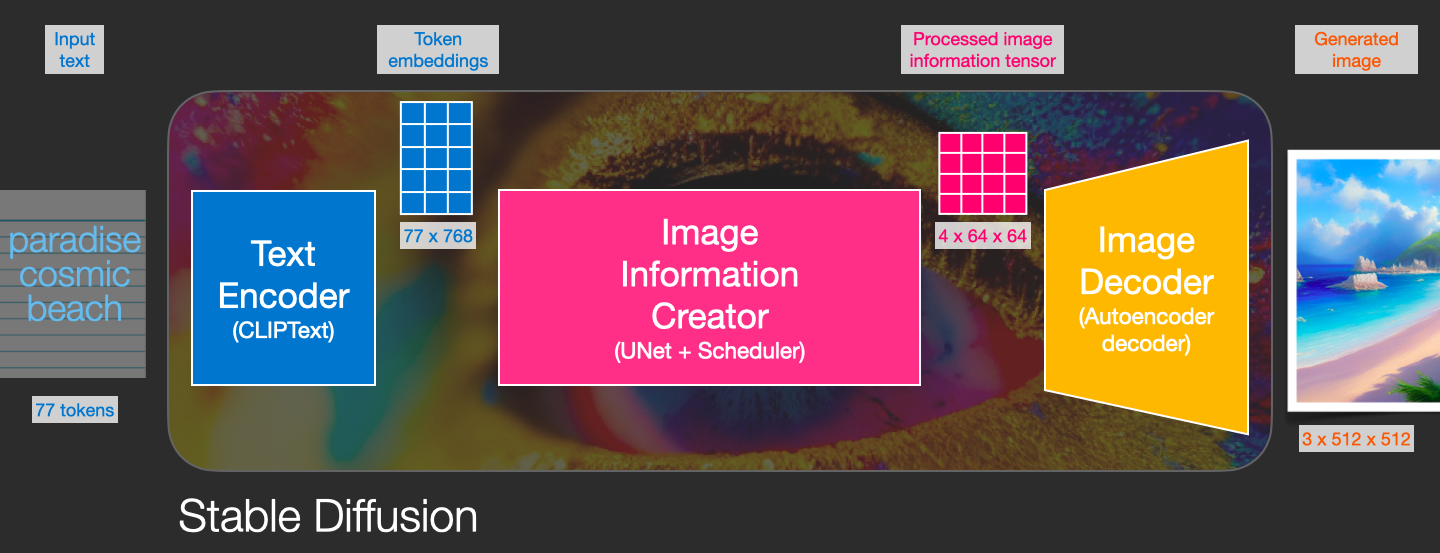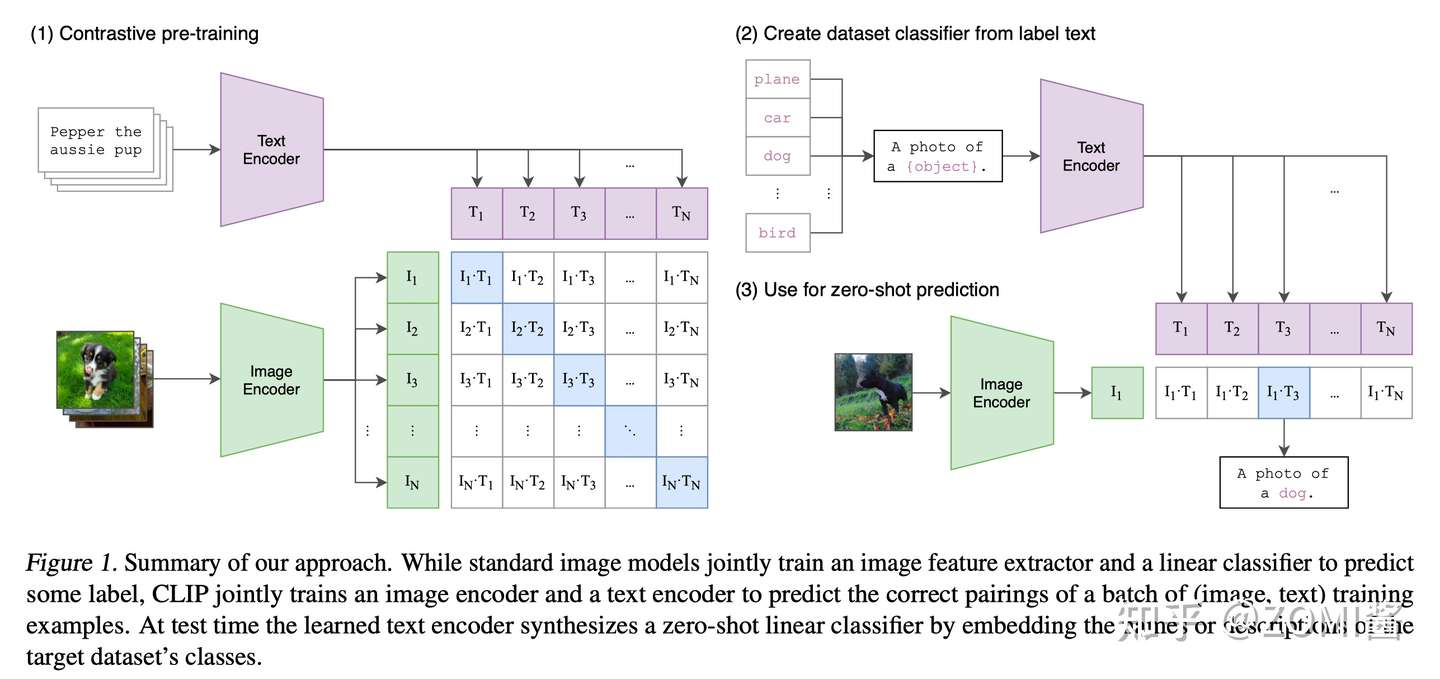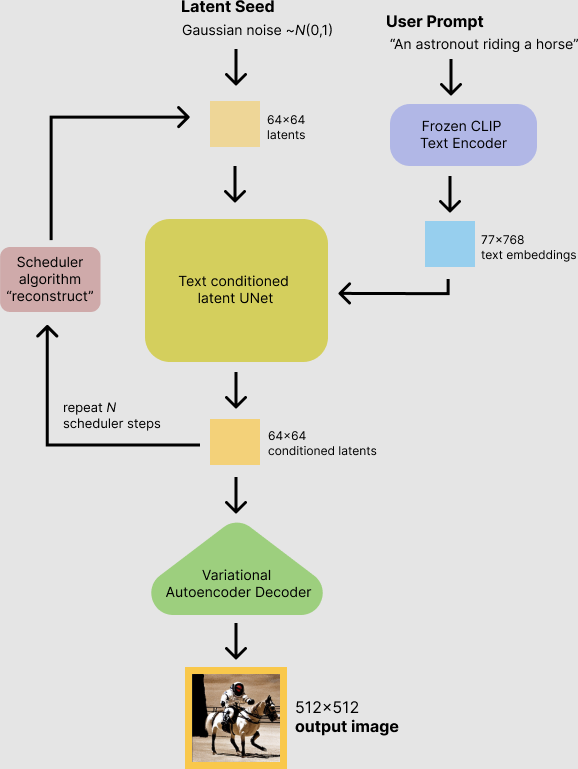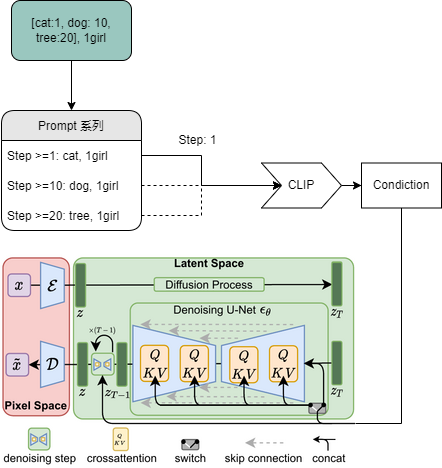# Help

## Explanation of terms

Abbreviations/terms Explanations
onehot a picture
LAION A library of image datasets, https://laion.ai
aug augmentaion, a way to get more datasets by cropping, flipping
ucg unconditional guidance
ML Machine Learning
Latent Space Latent Space
LDM Latent Diffusion Model

## How Stable Diffusion work

### Reasoning processinformation creator works entirely in the image information space (or latent space). This feature makes it faster than previous diffusion models that worked in pixel space. Technically, this component is composed of a UNet neural network and a scheduling algorithm.

Text Encoder

The parsing of the cues is processed by the Text Encoder/CLIP (token embedding), which is a key step in the translation of the cues to the AI.

The Text Encoder is responsible for converting the input hint into an embedding space that is understandable by U-Net. It is typically a simple transformer-based encoder that maps a sequence of input tokens into a sequence of latent text-embeddings.

Stable diffusion uses ClipText for text encoding. Input text, output 77 marker embedding vectors, each with 768 dimensions.

information creator

UNet + Scheduler (aka sampling algorithm) processes/disperses information step by step in the latent space.

It inputs text embeddings and a starting multidimensional array of noise (a structured list of numbers, also called a tensor) and outputs a processed array of information.

Image Decoder

Text Decoder draws a picture based on the information obtained from the information creator. It is run only once at the end of the process to generate the final image.

The autoencoder (VAE) model has two parts, an encoder and a decoder. The encoder is used to convert the image into a latent representation, which is used as input to the U-Net model. The decoder converts the latent representation back to an image.

In the inference process, the denoised latent image generated by the backward diffusion process is converted back to an image using the VAE decoder. In the inference process, we only need the VAE decoder.

Autoencoder Decoder (VAE) uses the processed information array to draw the decoder of the final image. Input processed information array (dimensions: (4, 64, 64)), output result image (dimensions: (3, 512, 512), i.e. (red/green/blue, width, height).

CLIP's workCLIP training graph from https://bbs.huaweicloud.com/blogs/371319

Process

The autoencoder used in Stable Diffusion has a reduction factor of 8. This means that an image of (4, 512, 512) is (4, 64, 64) in the potential space.

In the inference of a 512 x 512 image using stable diffusion, the model uses a seed and a text cue as input. The potential seed generates a random potential image of size 64 × 64, while the prompt enters the Text Encoder to be transformed into a text embedding of size 77 × 768 by CLIP's text encoder.

U-Net iteratively denoises the random Gaussian noise representation while conditioning on the text embedding. u-Net computes the denoised latent image representation through the sampling algorithm and outputs the noise residuals. After this step is repeated many times, the potential representation is decoded and output by the decoder of the Image Decoder's auto encoder.Extensions

What are Diffusion Models?

illustrated-stable-diffusion

Stable Diffusion

Introduction to Stable Diffusion

Stable Diffusion From Wikipedia

### Preprocessing for WebUi

Prompt_parser for WebUi Implemented with native WebUi for fading etc. functions.

The WebUi prompt syntax is converted into a prompt of the corresponding time, which is then passed on to Ai for processing by embedding.

Regarding the weighting implementation: the weighting increase usually takes up one prompt position.

For the implementation of the fade: at a given Step, the WebUi program replaces the corresponding prompt to achieve the fade effect.

And so on.

The whole process is shown in the diagramBy RcINS

### Math

diffusion_model

How diffusion models work: the math from scratch

## Term

### overfitting

Creating a model that matches the training data so closely that the model fails to make correct predictions on new data.

### convergence

Informally, often refers to a state reached during training in which training loss and validation loss change very little or not at all with each iteration after a certain number of iterations. In other words, a model reaches convergence when additional training on the current data will not improve the model. In deep learning, loss values sometimes stay constant or nearly so for many iterations before finally descending, temporarily producing a false sense of convergence.

### VAE

Variational autoencoders (VAEs) are a deep learning technique for learning latent representations. They have also been used to draw images, achieve state-of-the-art results in semi-supervised learning, as well as interpolate between sentences.2

### CFG

From 1 by Jon Stokes

This term stands for Classifier-Free Guidance Scale and is a measure of how close you want the model to stick to your prompt when looking for a related image to show you. (This is called “Prompt Guidance” in PlaygroundAI.) A Cfg Scale value of 0 will give you essentially a random image based on the seed, whereas a Cfg Scale of 20 (the maximum on SD) will give you the closest match to your prompt that the model can produce.

It’s worth trying to develop an intuition about this value in terms of latent space. The following analogy isn’t perfect, but it should give you a sense of what you’re doing:

Imagine your prompt is a flashlight with a variable-width beam, and you’re shining it onto the model’s latent space volume to highlight a particular region — your output image will be drawn from somewhere within that region, depending on the seed.

``````Dialing the Cfg Scale toward zero produces an extremely wide beam that highlights the entire latent space — your output could come from literally anywhere.

Dialing the Cfg Scale toward 20 produces a beam so narrow that at the extreme it turns into a laser pointer that illuminates a single point in latent space.
``````

Relate Paper

### Loss functions

In the context of an optimization algorithm, the function used to evaluate a candidate solution (i.e. a set of weights) is referred to as the objective function.4

Typically, with neural networks, we seek to minimize the error. As such, the objective function is often referred to as a cost function or a loss function and the value calculated by the loss function is referred to as simply “loss.”

### latent space

A representation of compressed data, where similar data points are spatially closer together. 5

For a explanation of latent space, please read Understanding latent space in machine learning.

### loss

A measure of how far a model's predictions are from its label. Or, to phrase it more pessimistically, a measure of how bad the model is. To determine this value, a model must define a loss function. For example, linear regression models typically use mean squared error for a loss function, while logistic regression models use Log Loss.6

### Hyperparameter

A parameter of a machine learning algorithm. Examples include the number of trees to learn in a decision forest or the step size in a gradient descent algorithm. Values of Hyperparameters are set before training the model and govern the process of finding the parameters of the prediction function, for example, the comparison points in a decision tree or the weights in a linear regression model. For more information, see the Hyperparameter article on Wikipedia.

### Pipeline

All of the operations needed to fit a model to a data set. A pipeline consists of data import, transformation, featurization, and learning steps. Once a pipeline is trained, it turns into a model.7

### epoch

A full training pass over the entire data set such that each example has been seen once. Thus, an epoch represents N/batch size training iterations, where N is the total number of examples.6

### batch size

The number of examples in a batch. For example, the batch size of SGD is 1, while the batch size of a mini-batch is usually between 10 and 1000. Batch size is usually fixed during training and inference; however, TensorFlow does permit dynamic batch sizes.6

### iteration

A single update of a model's weights during training. An iteration consists of computing the gradients of the parameters with respect to the loss on a single batch of data.6

### Tensor

The primary data structure in TensorFlow programs. Tensors are N-dimensional (where N could be very large) data structures, most commonly scalars, vectors, or matrices. The elements of a Tensor can hold integer, floating-point, or string values.

### checkpoint

Data that captures the state of the variables of a model at a particular time. Checkpoints enable exporting model weights, as well as performing training across multiple sessions. Checkpoints also enable training to continue past errors (for example, job preemption). Note that the graph itself is not included in a checkpoint.6

### embeddings

A categorical feature represented as a continuous-valued feature. Typically, an embedding is a translation of a high-dimensional vector into a low-dimensional space. For example, you can represent the words in an English sentence in either of the following two ways:

``````As a million-element (high-dimensional) sparse vector in which all elements are integers. Each cell in the vector represents a separate English word; the value in a cell represents the number of times that word appears in a sentence. Since a single English sentence is unlikely to contain more than 50 words, nearly every cell in the vector will contain a 0. The few cells that aren't 0 will contain a low integer (usually 1) representing the number of times that word appeared in the sentence.
As a several-hundred-element (low-dimensional) dense vector in which each element holds a floating-point value between 0 and 1. This is an embedding.
``````

In TensorFlow, embeddings are trained by backpropagating loss just like any other parameter in a neural network.6

### Active Function

A function (for example, ReLU or sigmoid) that takes in the weighted sum of all of the inputs from the previous layer and then generates and passes an output value (typically nonlinear) to the next layer.6

### weight

A coefficient for a feature in a linear model, or an edge in a deep network. The goal of training a linear model is to determine the ideal weight for each feature. If a weight is 0, then its corresponding feature does not contribute to the model.6

### ENSD

ENSD is the eta noise seed delta, which changes the seed.

NAI uses 31337

### CLIP

CLIP is a very advanced neural network that transforms your prompt text into a numerical representation. Neural networks work very well with this numerical representation and that's why devs of SD chose CLIP as one of 3 models involved in Stable Diffusion's method of producing images. As CLIP is a neural network, it means that it has a lot of layers. Your prompt is digitized in a simple way, and then fed through layers. You get numerical representation of the prompt after the 1st layer, you feed that into the second layer, you feed the result of that into third, etc, until you get to the last layer, and that's the output of CLIP that is used in Stable Diffusion. This is the slider value of 1. But you can stop early, and use the output of the next to last layer - that's slider value of 2. The earlier you stop, the less layers of neural network have worked on the prompt.

https://github.com/AUTOMATIC1111/stable-diffusion-webui/wiki/Features#ignore-last-layers-of-clip-model

WebUi uses the clip-interrogator project, which combines the blip and clip projects to greatly optimise the image to text process. blip is responsible for interpreting the text from the original image, while clip is responsible for interpreting the description of the new image suitable for creation.

### CUDA

With CUDA technology, the graphics card can be simulated as a PhysX physics acceleration chip.

Last update: April 15, 2023 03:42:26
Created: October 13, 2022 10:24:49